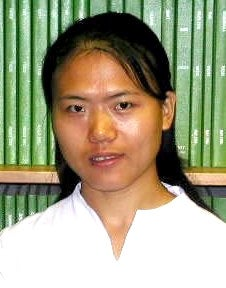# Chuili SunResume
Education
 Ph.D., Texas A&M University, Texas (2006) M.S., Tsinghua University, Beijing, China (2002) B.S., Tsinghua University, Beijing, China (1999)
Research Interests

The objective of process control is to maintain a desired level of product quality and safety while making the process more economical. Because these goals apply to a variety of industries, process control systems are used in facilities for the production of chemicals, pulp and paper, metals, food, pharmaceuticals, and microelectronics. While the methods of production vary from industry to industry, the principles of process control are generic in nature and can be universally applied, regardless of the size of the plant.
Process controllers that are based explicitly on dynamic mathematical models (i.e., sets of differential equations) have become increasingly popular in the chemical process industry. This is due to several reasons, the most important being that highly accurate models can now be solved with modern dynamic simulators and powerful optimization algorithms. However, computational requirements grow with the complexity of the models. Many rigorous dynamic models require too much computation time to be useful for real-time model based controllers. Real-time control is important, however, because the action of the controller must be computed in a time span that is faster than the dominant process time constant. Because control calculations for techniques such as linear model predictive control scale according to n3 (n = number of state variables), model reduction is a promising avenue for real-time implementation.
We have developed a novel strategy to nonlinear model reduction that includes system analysis, nonlinearity quantification, and model reduction. The advantages of this new approach are that the procedure is applicable to any kind of nonlinear syste mand that several system properties can be determined within the same framework resulting in an efficient strategy for the analysis of nonlinear systems. In the first step, covariance matrices, which are an extension of linear gramians to any type of system, are computed. These covariance matrices are then balanced by a novel algorithm that can be applied to nonlinear systems, with no assumption about rank deficiency of the computed covariance matrices being required. Two new types of nonlinearity measures were introduced that are based upon the balanced covariance matrices. If the measures indicate that the model exhibits only a mild degree of nonlinearity then it is appropriate to work with the linearization of the model, which greatly simplifies on-line application of the model. However, if the model is found to be strongly nonlinear over the required operating region, the information contained in the covariance matrices can be further used for reduction of the nonlinear model. Two new model reduction procedures for nonlinear systems were introduced in our research. The main task of the model reduction procedure is to determine an invertible state transformation such that the states are ordered according to their importance for the input-output behavior of the system. The approaches are based upon a strong theoretical foundation, in that they reduce to well known facts from linear systems theory when applied to linear systems, while they allow for the flexibility required for nonlinear systems.
These covariance matrix based methods have been applied in simulation to a series of reactors, a distillation column, and a polymerization reactor. The procedures reduced the number of differential equations in the model as well as the computation time required for the solution of these models. We have also demonstrated that several model reduction procedures, which have been developed over the last 20 years and were assumed to be independent from one another, form special cases of the procedure developed in my dissertation.
While these procedures were developed with the control of nonlinear systems in mind, they are applicable to any nonlinear system regardless of its use and can elucidate several of the properties exhibited by nonlinear systems.